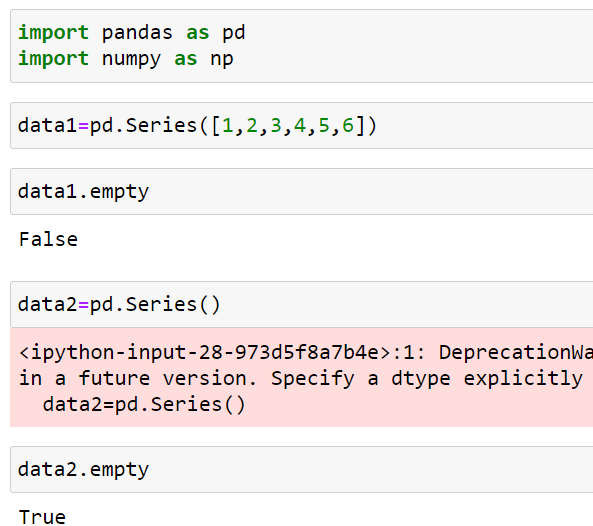top of page
Search

# Pandas Part 2

## Series

• Series is a one-dimensional labeled array

• We can think series as a single column or a row

• Elements inside the series has a label (index) to it index starts from 0

• The axis label collectively called index

• Series are immutable means once a series is created we cannot change its size

• Homogenous data means all the elements inside the series should be of same data type

• But values are mutable

## How To Create A Series Data Structure

• A series can be created by using the Series() constructor

` First import pandas  `

Example 1** as keyword is used for aliasing (temporary name )

### Parameters of Series Constructor

• Data - which data to use to construct a series .

Data can be a list, numpy array, tuple, dictionaries , constant value etc..

• index - axis labels

Default index is np.arange(len(data))

We can give our own index label .But the index value should be unique and it should be of same length of data

• dtype - data type for the data

### Creating Series Data Structure By Using Above Parameters

• Data

A series can be created using various data like array ,list, Dictionaries etc..

Example 2Example 3Example 4Example 5Example 6• Index

Default index will be np.arange(len(data)).But we can change the default index by using index parameter. Index should be unique

Example 7• dtype

we can use dtype parameter for changing the data type of the input data

can use numpy datatypes or python data types

Example 8## Series Attributes

• axes

Returns axis label

Example 8• dtype

Returns the data type of the elements inside the series

Example 9• empty

Checks whether series is empty or not .If empty returns True or False

Example 10• size

Returns the no of elements inside the series

Example 11• values

Returns the series as ndarray (use when we need to convert series to ndarray)

Example 12• ndim

Returns rank of the array (Series will always be 1 dimensional array)

Example 13## Accessing Data (Elements) From Series

• Elements in the series can be accessed by using [] (similar to accessing elements in list or ndarray)

• We can also use negative indexing

Example 14• Default index will be range(len(object))

• If index parameter used we can use the specified index to access the element

Example 15• We can also use slicing to access elements from the series (similar to list,ndarrays)

• Note ending index not included

• We can also use negative indexingExample 16## DataFrame

• DataFrame is a two-dimensional labeled array

• We can think DataFrame as a table which contains rows and columns

• Elements inside the DataFrame has a label (rowindex,column index) to it index starts from 0

• The axis labels collectively called row index and column index

• DataFrame are mutable means once a DataFrame is created we can change its shape

• Homogenous data column wise means all the elements should be of same data type (columns)

• Heterogenous data row wise means different elements (rows)

• Values are mutable

• DataFrame can be considered as a container holding series data structures (each column inside a DataFrame can be considered as a series data structure.That's why columns have homogenous data and rows have heterogenous data)

## How To Create A Series Data Structure

• A DataFrame can be created by using the DataFrame() constructor

Example 17** as keyword is used for aliasing (temporary name )

### Parameters of DataFrame Constructor

• Data - which data to use to construct a series .

Data can be a list, numpy array, tuple, dictionaries , constant value etc..

• index - axis labels (Row axis labels)

Default index is np.arange(len(data))

We can give our own index label .But the index value should be unique and it should be of same length of data

• dtype - data type for the data

• columns - axis labels (Column axis labels)

Default index is np.arange(len(data))

We can give our own index label .But the index value should be unique and it should be of same length of data

### Creating Data Frame Data Structure By Using Above Parameters

• Data

Data can be a list, numpy array, tuple, dictionaries , constant value etc..

Example 18Example 19Example 20Example 21• index

As Data Frame is a 2 dimensional data structure we can provide labels for both rows and columns so index is used for row axis labels (Row axis labels)

Example 22• dtype

we can change the data type for the elements by using dtype parameters

Example 23• columns

As Data Frame is a 2 dimensional data structure we can provide labels for both rows and columns so columns is used for column axis labels (Row axis labels)

Example 24Example 25** when using dictionary the key will behave as column name so if we use columns argument it will check whether the defined column is there or not if there it will return only that particular column values or else it will return and empty dictionaries or elements will be filled with nan with that particular column name